# Computer arithmetic in computer architecture

7 de Mar de 2017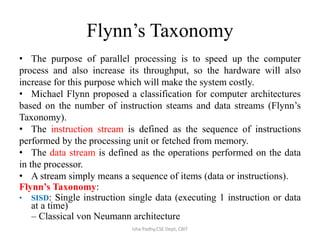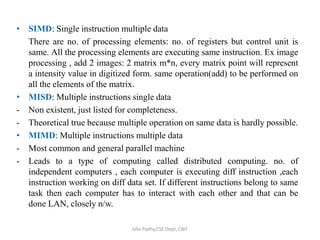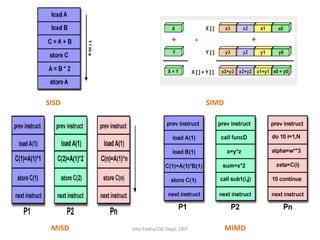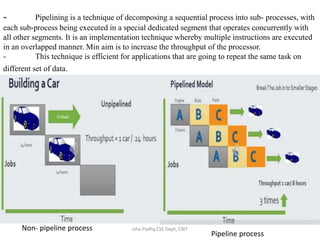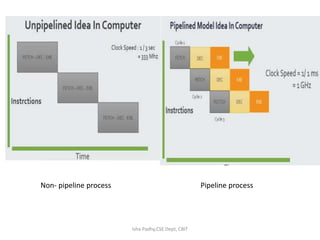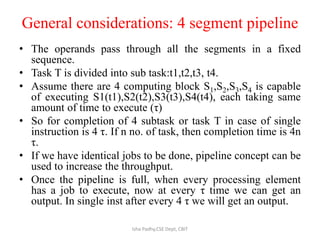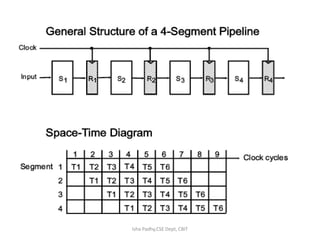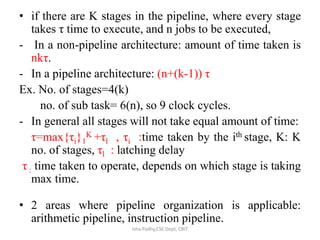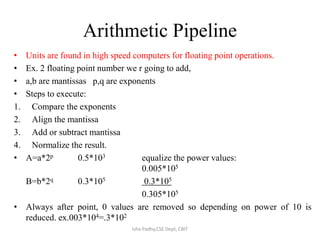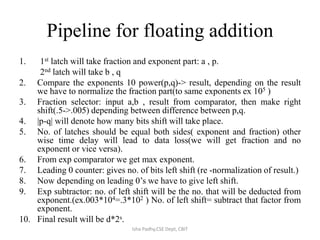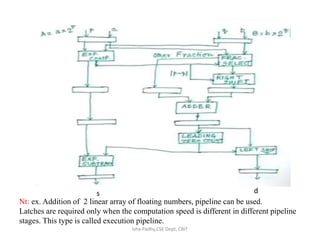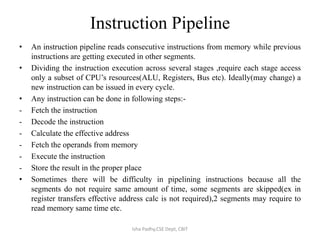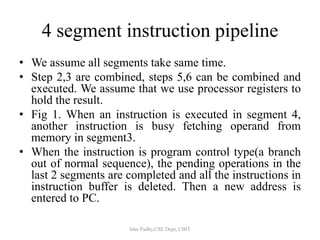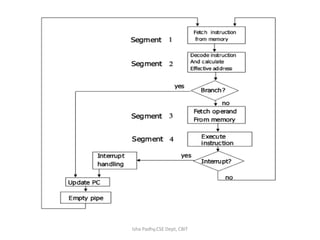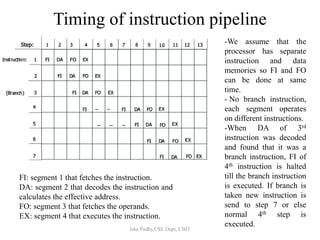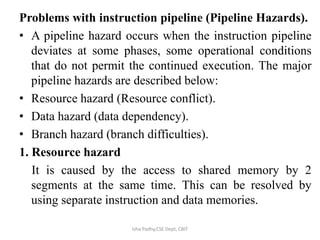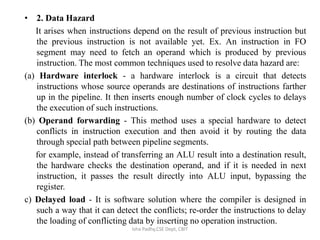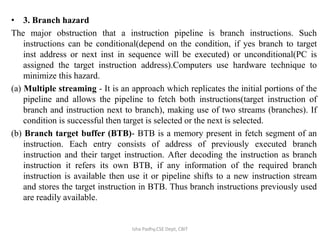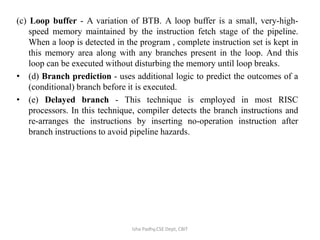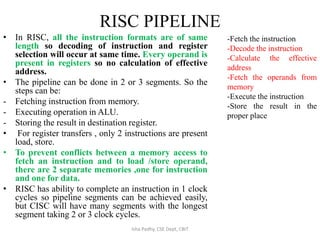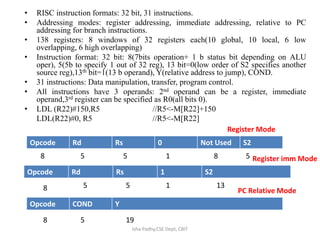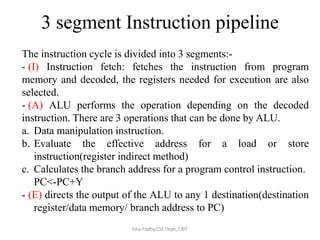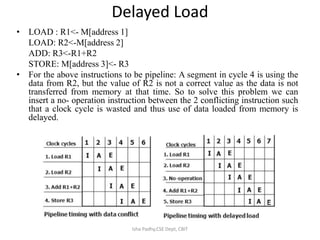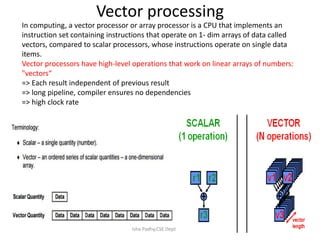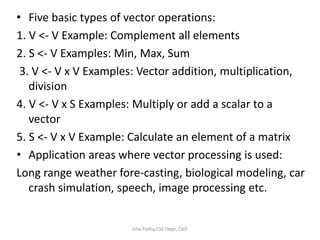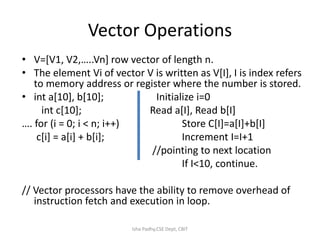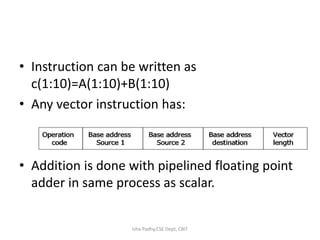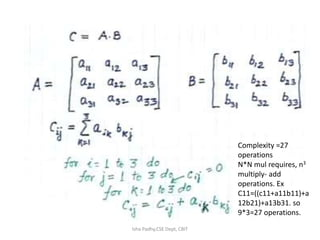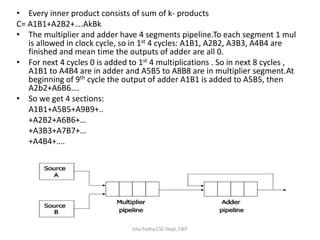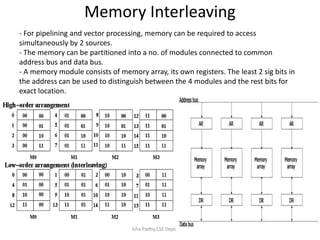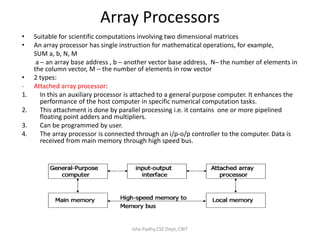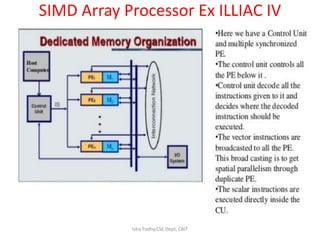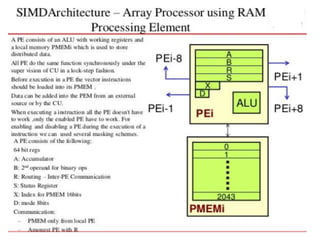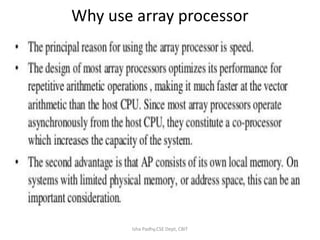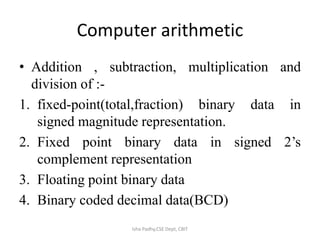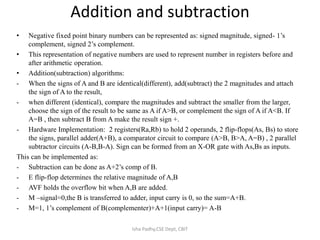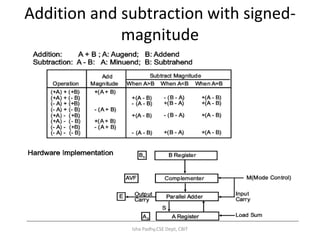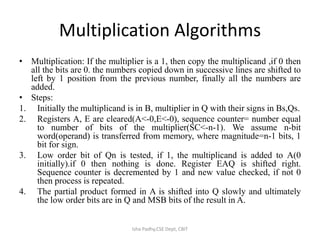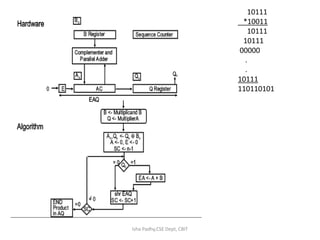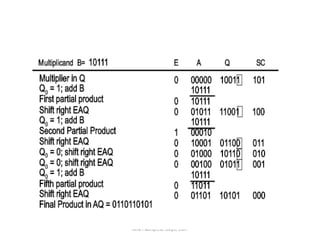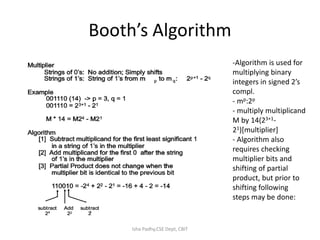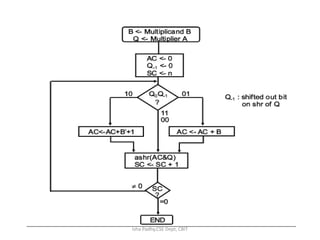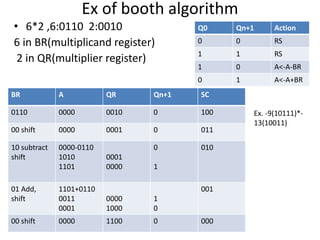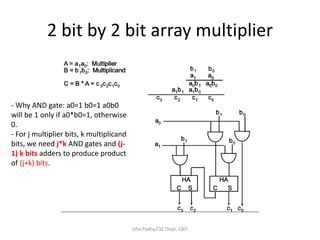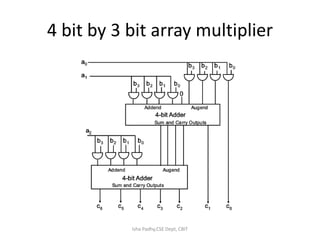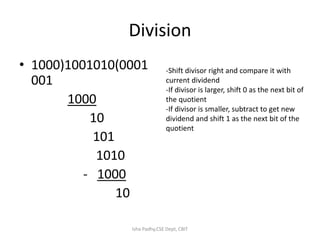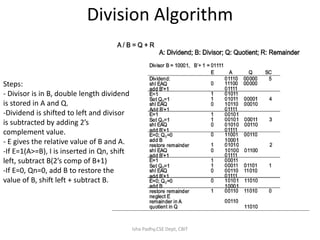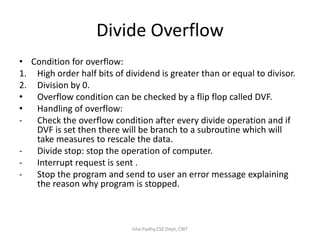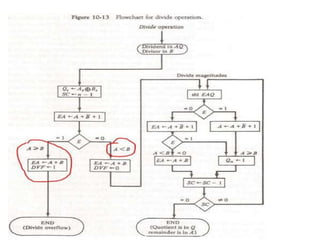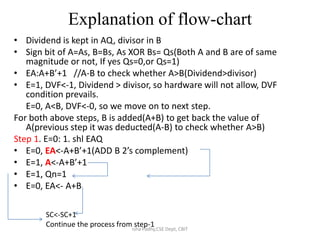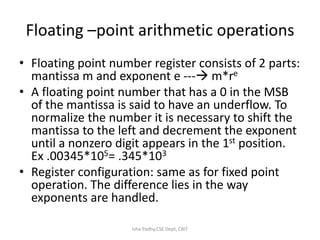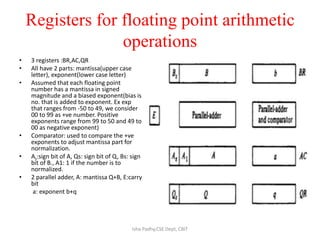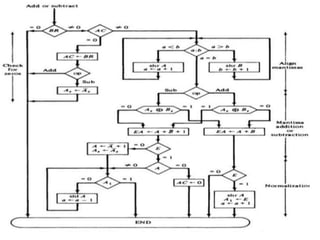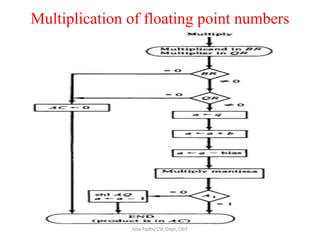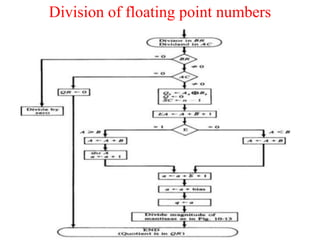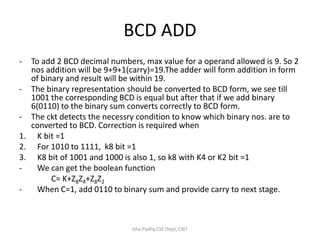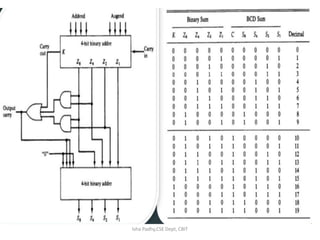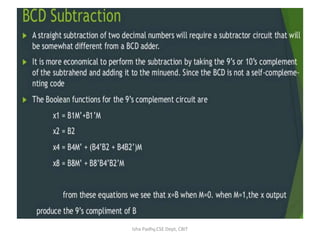1 de 58

### Computer arithmetic in computer architecture

• 1. Flynn’s Taxonomy • The purpose of parallel processing is to speed up the computer process and also increase its throughput, so the hardware will also increase for this purpose which will make the system costly. • Michael Flynn proposed a classification for computer architectures based on the number of instruction steams and data streams (Flynn’s Taxonomy). • The instruction stream is defined as the sequence of instructions performed by the processing unit or fetched from memory. • The data stream is defined as the operations performed on the data in the processor. • A stream simply means a sequence of items (data or instructions). Flynn’s Taxonomy: • SISD: Single instruction single data (executing 1 instruction or data at a time) – Classical von Neumann architecture Isha Padhy,CSE Dept, CBIT
• 2. • SIMD: Single instruction multiple data There are no. of processing elements: no. of registers but control unit is same. All the processing elements are executing same instruction. Ex image processing , add 2 images: 2 matrix m*n, every matrix point will represent a intensity value in digitized form. same operation(add) to be performed on all the elements of the matrix. • MISD: Multiple instructions single data - Non existent, just listed for completeness. - Theoretical true because multiple operation on same data is hardly possible. • MIMD: Multiple instructions multiple data - Most common and general parallel machine - Leads to a type of computing called distributed computing. no. of independent computers , each computer is executing diff instruction ,each instruction working on diff data set. If different instructions belong to same task then each computer has to interact with each other and that can be done LAN, closely n/w. Isha Padhy,CSE Dept, CBIT
• 3. SISD SIMD MISD MIMDIsha Padhy,CSE Dept, CBIT
• 4. Non- pipeline process Pipeline process - Pipelining is a technique of decomposing a sequential process into sub- processes, with each sub-process being executed in a special dedicated segment that operates concurrently with all other segments. It is an implementation technique whereby multiple instructions are executed in an overlapped manner. Min aim is to increase the throughput of the processor. - This technique is efficient for applications that are going to repeat the same task on different set of data. Isha Padhy,CSE Dept, CBIT
• 5. Non- pipeline process Pipeline process Isha Padhy,CSE Dept, CBIT
• 6. General considerations: 4 segment pipeline • The operands pass through all the segments in a fixed sequence. • Task T is divided into sub task:t1,t2,t3, t4. • Assume there are 4 computing block S1,S2,S3,S4 is capable of executing S1(t1),S2(t2),S3(t3),S4(t4), each taking same amount of time to execute (τ) • So for completion of 4 subtask or task T in case of single instruction is 4 τ. If n no. of task, then completion time is 4n τ. • If we have identical jobs to be done, pipeline concept can be used to increase the throughput. • Once the pipeline is full, when every processing element has a job to execute, now at every τ time we can get an output. In single inst after every 4 τ we will get an output. Isha Padhy,CSE Dept, CBIT
• 8. • if there are K stages in the pipeline, where every stage takes τ time to execute, and n jobs to be executed, - In a non-pipeline architecture: amount of time taken is nkτ. - In a pipeline architecture: (n+(k-1)) τ Ex. No. of stages=4(k) no. of sub task= 6(n), so 9 clock cycles. - In general all stages will not take equal amount of time: τ=max{τi}1 K +τl , τi :time taken by the ith stage, K: K no. of stages, τl : latching delay τ : time taken to operate, depends on which stage is taking max time. • 2 areas where pipeline organization is applicable: arithmetic pipeline, instruction pipeline. Isha Padhy,CSE Dept, CBIT
• 9. Arithmetic Pipeline • Units are found in high speed computers for floating point operations. • Ex. 2 floating point number we r going to add, • a,b are mantissas p,q are exponents • Steps to execute: 1. Compare the exponents 2. Align the mantissa 3. Add or subtract mantissa 4. Normalize the result. • A=a*2p 0.5*103 equalize the power values: 0.005*105 B=b*2q 0.3*105 0.3*105 0.305*105 • Always after point, 0 values are removed so depending on power of 10 is reduced. ex.003*104=.3*102 Isha Padhy,CSE Dept, CBIT
• 10. Pipeline for floating addition 1. 1st latch will take fraction and exponent part: a , p. 2nd latch will take b , q 2. Compare the exponents 10 power(p,q)-> result, depending on the result we have to normalize the fraction part(to same exponents ex 105 ) 3. Fraction selector: input a,b , result from comparator, then make right shift(.5->.005) depending between difference between p,q. 4. |p-q| will denote how many bits shift will take place. 5. No. of latches should be equal both sides( exponent and fraction) other wise time delay will lead to data loss(we will get fraction and no exponent or vice versa). 6. From exp comparator we get max exponent. 7. Leading 0 counter: gives no. of bits left shift (re -normalization of result.) 8. Now depending on leading 0’s we have to give left shift. 9. Exp subtractor: no. of left shift will be the no. that will be deducted from exponent.(ex.003*104=.3*102 ) No. of left shift= subtract that factor from exponent. 10. Final result will be d*2s. Isha Padhy,CSE Dept, CBIT
• 11. Nt: ex. Addition of 2 linear array of floating numbers, pipeline can be used. Latches are required only when the computation speed is different in different pipeline stages. This type is called execution pipeline. s d Isha Padhy,CSE Dept, CBIT
• 12. Instruction Pipeline • An instruction pipeline reads consecutive instructions from memory while previous instructions are getting executed in other segments. • Dividing the instruction execution across several stages ,require each stage access only a subset of CPU’s resources(ALU, Registers, Bus etc). Ideally(may change) a new instruction can be issued in every cycle. • Any instruction can be done in following steps:- - Fetch the instruction - Decode the instruction - Calculate the effective address - Fetch the operands from memory - Execute the instruction - Store the result in the proper place • Sometimes there will be difficulty in pipelining instructions because all the segments do not require same amount of time, some segments are skipped(ex in register transfers effective address calc is not required),2 segments may require to read memory same time etc. Isha Padhy,CSE Dept, CBIT
• 13. 4 segment instruction pipeline • We assume all segments take same time. • Step 2,3 are combined, steps 5,6 can be combined and executed. We assume that we use processor registers to hold the result. • Fig 1. When an instruction is executed in segment 4, another instruction is busy fetching operand from memory in segment3. • When the instruction is program control type(a branch out of normal sequence), the pending operations in the last 2 segments are completed and all the instructions in instruction buffer is deleted. Then a new address is entered to PC. Isha Padhy,CSE Dept, CBIT
• 15. Timing of instruction pipeline Isha Padhy,CSE Dept, CBIT -We assume that the processor has separate instruction and data memories so FI and FO can be done at same time. - No branch instruction, each segment operates on different instructions. -When DA of 3rd instruction was decoded and found that it was a branch instruction, FI of 4th instruction is halted till the branch instruction is executed. If branch is taken new instruction is send to step 7 or else normal 4th step is executed. FI: segment 1 that fetches the instruction. DA: segment 2 that decodes the instruction and calculates the effective address. FO: segment 3 that fetches the operands. EX: segment 4 that executes the instruction.
• 16. Problems with instruction pipeline (Pipeline Hazards). • A pipeline hazard occurs when the instruction pipeline deviates at some phases, some operational conditions that do not permit the continued execution. The major pipeline hazards are described below: • Resource hazard (Resource conflict). • Data hazard (data dependency). • Branch hazard (branch difficulties). 1. Resource hazard It is caused by the access to shared memory by 2 segments at the same time. This can be resolved by using separate instruction and data memories. Isha Padhy,CSE Dept, CBIT
• 17. • 2. Data Hazard It arises when instructions depend on the result of previous instruction but the previous instruction is not available yet. Ex. An instruction in FO segment may need to fetch an operand which is produced by previous instruction. The most common techniques used to resolve data hazard are: (a) Hardware interlock - a hardware interlock is a circuit that detects instructions whose source operands are destinations of instructions farther up in the pipeline. It then inserts enough number of clock cycles to delays the execution of such instructions. (b) Operand forwarding - This method uses a special hardware to detect conflicts in instruction execution and then avoid it by routing the data through special path between pipeline segments. for example, instead of transferring an ALU result into a destination result, the hardware checks the destination operand, and if it is needed in next instruction, it passes the result directly into ALU input, bypassing the register. c) Delayed load - It is software solution where the compiler is designed in such a way that it can detect the conflicts; re-order the instructions to delay the loading of conflicting data by inserting no operation instruction. Isha Padhy,CSE Dept, CBIT
• 18. • 3. Branch hazard The major obstruction that a instruction pipeline is branch instructions. Such instructions can be conditional(depend on the condition, if yes branch to target inst address or next inst in sequence will be executed) or unconditional(PC is assigned the target instruction address).Computers use hardware technique to minimize this hazard. (a) Multiple streaming - It is an approach which replicates the initial portions of the pipeline and allows the pipeline to fetch both instructions(target instruction of branch and instruction next to branch), making use of two streams (branches). If condition is successful then target is selected or the next is selected. (b) Branch target buffer (BTB)- BTB is a memory present in fetch segment of an instruction. Each entry consists of address of previously executed branch instruction and their target instruction. After decoding the instruction as branch instruction it refers its own BTB, if any information of the required branch instruction is available then use it or pipeline shifts to a new instruction stream and stores the target instruction in BTB. Thus branch instructions previously used are readily available. Isha Padhy,CSE Dept, CBIT
• 19. (c) Loop buffer - A variation of BTB. A loop buffer is a small, very-high- speed memory maintained by the instruction fetch stage of the pipeline. When a loop is detected in the program , complete instruction set is kept in this memory area along with any branches present in the loop. And this loop can be executed without disturbing the memory until loop breaks. • (d) Branch prediction - uses additional logic to predict the outcomes of a (conditional) branch before it is executed. • (e) Delayed branch - This technique is employed in most RISC processors. In this technique, compiler detects the branch instructions and re-arranges the instructions by inserting no-operation instruction after branch instructions to avoid pipeline hazards. Isha Padhy,CSE Dept, CBIT
• 20. RISC PIPELINE • In RISC, all the instruction formats are of same length so decoding of instruction and register selection will occur at same time. Every operand is present in registers so no calculation of effective address. • The pipeline can be done in 2 or 3 segments. So the steps can be: - Fetching instruction from memory. - Executing operation in ALU. - Storing the result in destination register. • For register transfers , only 2 instructions are present load, store. • To prevent conflicts between a memory access to fetch an instruction and to load /store operand, there are 2 separate memories ,one for instruction and one for data. • RISC has ability to complete an instruction in 1 clock cycles so pipeline segments can be achieved easily, but CISC will have many segments with the longest segment taking 2 or 3 clock cycles. Isha Padhy, CSE Dept, CBIT -Fetch the instruction -Decode the instruction -Calculate the effective address -Fetch the operands from memory -Execute the instruction -Store the result in the proper place
• 21. • RISC instruction formats: 32 bit, 31 instructions. • Addressing modes: register addressing, immediate addressing, relative to PC addressing for branch instructions. • 138 registers: 8 windows of 32 registers each(10 global, 10 local, 6 low overlapping, 6 high overlapping) • Instruction format: 32 bit: 8(7bits operation+ 1 b status bit depending on ALU oper), 5(5b to specify 1 out of 32 reg), 13 bit=0(low order of S2 specifies another source reg),13th bit=1(13 b operand), Y(relative address to jump), COND. • 31 instructions: Data manipulation, transfer, program control. • All instructions have 3 operands: 2nd operand can be a register, immediate operand,3rd register can be specified as R0(all bits 0). • LDL (R22)#150,R5 //R5<-M[R22]+150 LDL(R22)#0, R5 //R5<-M[R22] Isha Padhy,CSE Dept, CBIT Opcode Rd Rs 0 Not Used S2 Opcode Rd Rs 1 S2 Opcode COND Y 8 5 5 1 8 5 8 5 5 1 13 8 5 19 Register Mode Register imm Mode PC Relative Mode
• 22. 3 segment Instruction pipeline Isha Padhy,CSE Dept, CBIT The instruction cycle is divided into 3 segments:- - (I) Instruction fetch: fetches the instruction from program memory and decoded, the registers needed for execution are also selected. - (A) ALU performs the operation depending on the decoded instruction. There are 3 operations that can be done by ALU. a. Data manipulation instruction. b. Evaluate the effective address for a load or store instruction(register indirect method) c. Calculates the branch address for a program control instruction. PC<-PC+Y - (E) directs the output of the ALU to any 1 destination(destination register/data memory/ branch address to PC)
• 23. Delayed Load • LOAD : R1<- M[address 1] LOAD: R2<-M[address 2] ADD: R3<-R1+R2 STORE: M[address 3]<- R3 • For the above instructions to be pipeline: A segment in cycle 4 is using the data from R2, but the value of R2 is not a correct value as the data is not transferred from memory at that time. So to solve this problem we can insert a no- operation instruction between the 2 conflicting instruction such that a clock cycle is wasted and thus use of data loaded from memory is delayed. Isha Padhy,CSE Dept, CBIT
• 24. Vector processing Isha Padhy,CSE Dept, CBIT In computing, a vector processor or array processor is a CPU that implements an instruction set containing instructions that operate on 1- dim arrays of data called vectors, compared to scalar processors, whose instructions operate on single data items. Vector processors have high-level operations that work on linear arrays of numbers: "vectors“ => Each result independent of previous result => long pipeline, compiler ensures no dependencies => high clock rate
• 25. • Five basic types of vector operations: 1. V <- V Example: Complement all elements 2. S <- V Examples: Min, Max, Sum 3. V <- V x V Examples: Vector addition, multiplication, division 4. V <- V x S Examples: Multiply or add a scalar to a vector 5. S <- V x V Example: Calculate an element of a matrix • Application areas where vector processing is used: Long range weather fore-casting, biological modeling, car crash simulation, speech, image processing etc. Isha Padhy,CSE Dept, CBIT
• 26. Vector Operations Isha Padhy,CSE Dept, CBIT • V=[V1, V2,…..Vn] row vector of length n. • The element Vi of vector V is written as V[I], I is index refers to memory address or register where the number is stored. • int a, b; Initialize i=0 int c; Read a[I], Read b[I] …. for (i = 0; i < n; i++) Store C[I]=a[I]+b[I] c[i] = a[i] + b[i]; Increment I=I+1 //pointing to next location If I<10, continue. // Vector processors have the ability to remove overhead of instruction fetch and execution in loop.
• 27. • Instruction can be written as c(1:10)=A(1:10)+B(1:10) • Any vector instruction has: • Addition is done with pipelined floating point adder in same process as scalar. Isha Padhy,CSE Dept, CBIT
• 28. Isha Padhy,CSE Dept, CBIT Complexity =27 operations N*N mul requires, n3 multiply- add operations. Ex C11=((c11+a11b11)+a 12b21)+a13b31. so 9*3=27 operations.
• 29. Isha Padhy,CSE Dept, CBIT • Every inner product consists of sum of k- products C= A1B1+A2B2+….AkBk • The multiplier and adder have 4 segments pipeline.To each segment 1 mul is allowed in clock cycle, so in 1st 4 cycles: A1B1, A2B2, A3B3, A4B4 are finished and mean time the outputs of adder are all 0. • For next 4 cycles 0 is added to 1st 4 multiplications . So in next 8 cycles , A1B1 to A4B4 are in adder and A5B5 to A8B8 are in multiplier segment.At beginning of 9th cycle the output of adder A1B1 is added to A5B5, then A2b2+A6B6…. • So we get 4 sections: A1B1+A5B5+A9B9+.. +A2B2+A6B6+… +A3B3+A7B7+… +A4B4+….
• 30. Memory Interleaving Isha Padhy,CSE Dept, CBIT - For pipelining and vector processing, memory can be required to access simultaneously by 2 sources. - The memory can be partitioned into a no. of modules connected to common address bus and data bus. - A memory module consists of memory array, its own registers. The least 2 sig bits in the address can be used to distinguish between the 4 modules and the rest bits for exact location.
• 31. Array Processors • Suitable for scientific computations involving two dimensional matrices • An array processor has single instruction for mathematical operations, for example, SUM a, b, N, M a ─ an array base address , b ─ another vector base address, N─ the number of elements in the column vector, M ─ the number of elements in row vector • 2 types: - Attached array processor: 1. In this an auxiliary processor is attached to a general purpose computer. It enhances the performance of the host computer in specific numerical computation tasks. 2. This attachment is done by parallel processing i.e. it contains one or more pipelined floating point adders and multipliers. 3. Can be programmed by user. 4. The array processor is connected through an i/p-o/p controller to the computer. Data is received from main memory through high speed bus. Isha Padhy,CSE Dept, CBIT
• 32. SIMD Array Processor Ex ILLIAC IV Isha Padhy,CSE Dept, CBIT
• 34. Why use array processor Isha Padhy,CSE Dept, CBIT
• 35. Computer arithmetic • Addition , subtraction, multiplication and division of :- 1. fixed-point(total,fraction) binary data in signed magnitude representation. 2. Fixed point binary data in signed 2’s complement representation 3. Floating point binary data 4. Binary coded decimal data(BCD) Isha Padhy,CSE Dept, CBIT
• 36. Addition and subtraction • Negative fixed point binary numbers can be represented as: signed magnitude, signed- 1’s complement, signed 2’s complement. • This representation of negative numbers are used to represent number in registers before and after arithmetic operation. • Addition(subtraction) algorithms: - When the signs of A and B are identical(different), add(subtract) the 2 magnitudes and attach the sign of A to the result, - when different (identical), compare the magnitudes and subtract the smaller from the larger, choose the sign of the result to be same as A if A>B, or complement the sign of A if A<B. If A=B , then subtract B from A make the result sign +. - Hardware Implementation: 2 registers(Ra,Rb) to hold 2 operands, 2 flip-flops(As, Bs) to store the signs, parallel adder(A+B), a comparator circuit to compare (A>B, B>A, A=B) , 2 parallel subtractor circuits (A-B,B-A). Sign can be formed from an X-OR gate with As,Bs as inputs. This can be implemented as: - Subtraction can be done as A+2’s comp of B. - E flip-flop determines the relative magnitude of A,B - AVF holds the overflow bit when A,B are added. - M –signal=0,the B is transferred to adder, input carry is 0, so the sum=A+B. - M=1, 1’s complement of B(complementer)+A+1(input carry)= A-B Isha Padhy,CSE Dept, CBIT
• 37. Addition and subtraction with signed- magnitude Isha Padhy,CSE Dept, CBIT
• 38. Multiplication Algorithms • Multiplication: If the multiplier is a 1, then copy the multiplicand ,if 0 then all the bits are 0. the numbers copied down in successive lines are shifted to left by 1 position from the previous number, finally all the numbers are added. • Steps: 1. Initially the multiplicand is in B, multiplier in Q with their signs in Bs,Qs. 2. Registers A, E are cleared(A<-0,E<-0), sequence counter= number equal to number of bits of the multiplier(SC<-n-1). We assume n-bit word(operand) is transferred from memory, where magnitude=n-1 bits, 1 bit for sign. 3. Low order bit of Qn is tested, if 1, the multiplicand is added to A(0 initially).if 0 then nothing is done. Register EAQ is shifted right. Sequence counter is decremented by 1 and new value checked, if not 0 then process is repeated. 4. The partial product formed in A is shifted into Q slowly and ultimately the low order bits are in Q and MSB bits of the result in A. Isha Padhy,CSE Dept, CBIT
• 39. Isha Padhy,CSE Dept, CBIT 10111 *10011 10111 10111 00000 . . 10111 110110101
• 41. Booth’s Algorithm Isha Padhy,CSE Dept, CBIT -Algorithm is used for multiplying binary integers in signed 2’s compl. - mp:2p - multiply multiplicand M by 14(23+1- 21)[multiplier] - Algorithm also requires checking multiplier bits and shifting of partial product, but prior to shifting following steps may be done:
• 43. Ex of booth algorithm • 6*2 ,6:0110 2:0010 6 in BR(multiplicand register) 2 in QR(multiplier register) Isha Padhy,CSE Dept, CBIT Q0 Qn+1 Action 0 0 RS 1 1 RS 1 0 A<-A-BR 0 1 A<-A+BR BR A QR Qn+1 SC 0110 0000 0010 0 100 00 shift 0000 0001 0 011 10 subtract shift 0000-0110 1010 1101 0001 0000 0 1 010 01 Add, shift 1101+0110 0011 0001 0000 1000 1 0 001 00 shift 0000 1100 0 000 Ex. -9(10111)*- 13(10011)
• 44. 2 bit by 2 bit array multiplier Isha Padhy,CSE Dept, CBIT - Why AND gate: a0=1 b0=1 a0b0 will be 1 only if a0*b0=1, otherwise 0. - For j multiplier bits, k multiplicand bits, we need j*k AND gates and (j- 1) k bits adders to produce product of (j+k) bits.
• 45. 4 bit by 3 bit array multiplier Isha Padhy,CSE Dept, CBIT
• 46. Division • 1000)1001010(0001 001 1000 10 101 1010 - 1000 10 Isha Padhy,CSE Dept, CBIT -Shift divisor right and compare it with current dividend -If divisor is larger, shift 0 as the next bit of the quotient -If divisor is smaller, subtract to get new dividend and shift 1 as the next bit of the quotient
• 47. Division Algorithm Isha Padhy,CSE Dept, CBIT Steps: - Divisor is in B, double length dividend is stored in A and Q. -Dividend is shifted to left and divisor is subtracted by adding 2’s complement value. - E gives the relative value of B and A. -If E=1(A>=B), I is inserted in Qn, shift left, subtract B(2’s comp of B+1) -If E=0, Qn=0, add B to restore the value of B, shift left + subtract B.
• 48. Divide Overflow • Condition for overflow: 1. High order half bits of dividend is greater than or equal to divisor. 2. Division by 0. • Overflow condition can be checked by a flip flop called DVF. • Handling of overflow: - Check the overflow condition after every divide operation and if DVF is set then there will be branch to a subroutine which will take measures to rescale the data. - Divide stop: stop the operation of computer. - Interrupt request is sent . - Stop the program and send to user an error message explaining the reason why program is stopped. Isha Padhy,CSE Dept, CBIT
• 50. Explanation of flow-chart • Dividend is kept in AQ, divisor in B • Sign bit of A=As, B=Bs, As XOR Bs= Qs(Both A and B are of same magnitude or not, If yes Qs=0,or Qs=1) • EA:A+B’+1 //A-B to check whether A>B(Dividend>divisor) • E=1, DVF<-1, Dividend > divisor, so hardware will not allow, DVF condition prevails. E=0, A<B, DVF<-0, so we move on to next step. For both above steps, B is added(A+B) to get back the value of A(previous step it was deducted(A-B) to check whether A>B) Step 1. E=0: 1. shl EAQ • E=0, EA<-A+B’+1(ADD B 2’s complement) • E=1, A<-A+B’+1 • E=1, Qn=1 • E=0, EA<- A+B Isha Padhy,CSE Dept, CBIT SC<-SC+1 Continue the process from step-1
• 51. Floating –point arithmetic operations • Floating point number register consists of 2 parts: mantissa m and exponent e --- m*re • A floating point number that has a 0 in the MSB of the mantissa is said to have an underflow. To normalize the number it is necessary to shift the mantissa to the left and decrement the exponent until a nonzero digit appears in the 1st position. Ex .00345*105= .345*103 • Register configuration: same as for fixed point operation. The difference lies in the way exponents are handled. Isha Padhy,CSE Dept, CBIT
• 52. Registers for floating point arithmetic operations • 3 registers :BR,AC,QR • All have 2 parts: mantissa(upper case letter), exponent(lower case letter) • Assumed that each floating point number has a mantissa in signed magnitude and a biased exponent(bias is no. that is added to exponent. Ex exp that ranges from -50 to 49, we consider 00 to 99 as +ve number. Positive exponents range from 99 to 50 and 49 to 00 as negative exponent) • Comparator: used to compare the +ve exponents to adjust mantissa part for normalization. • As:sign bit of A, Qs: sign bit of Q, Bs: sign bit of B., A1: 1 if the number is to normalized. • 2 parallel adder, A: mantissa Q+B, E:carry bit a: exponent b+q Isha Padhy,CSE Dept, CBIT